### IMO Shortlist 2012 problem G2

Kvaliteta:
Avg: 3,3
Težina:
Avg: 6,0
Let$ABCD$ be a cyclic quadrilateral whose diagonals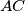$AC$ and$BD$ meet at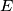$E$. The extensions of the sides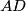$AD$ and$BC$ beyond$A$ and$B$ meet at$F$. Let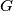$G$ be the point such that$ECGD$ is a parallelogram, and let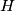$H$ be the image of$E$ under reflection in$AD$. Prove that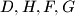$D,H,F,G$ are concyclic.
Izvor: Međunarodna matematička olimpijada, shortlist 2012